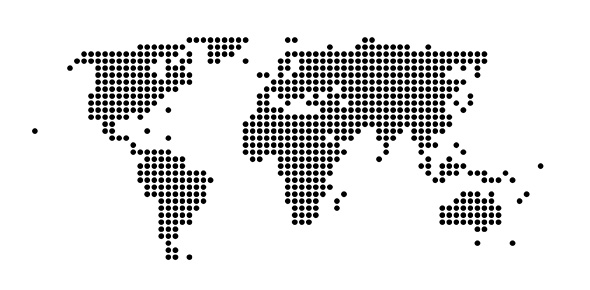# Map Scales

3 Questions | Total Attempts: 534SettingsThis is a pre-test before the lesson. My hypothesis is you are able to do these questions by pooling together your knowledge already learned through Geography and Mathematics to attempt these questions.

Related Topics
• 1.
Two bus-stops 800 m apart measures 2 cm on a map. The map scale is 1: n. Find the value of n.
• A.

400

• B.

2500

• C.

40000

• D.

400000

• E.

250000

• 2.
A hiking trail measures 8 cm on a 1: 50000 map. Calculate the actual distance of the hiking trail. Express your answer in terms of km.
• A.

4

• B.

6.25

• C.

400000

• D.

400

• E.

6250

• 3.
A school occupies a rectangular plot of land that measures 8 cm by 3 cm on a map where a 100m running track measures 2 cm. Calculate the actual floor area occupied by the school in terms of km2.
• A.

2400

• B.

1200

• C.

1.2

• D.

0.06

• E.

600